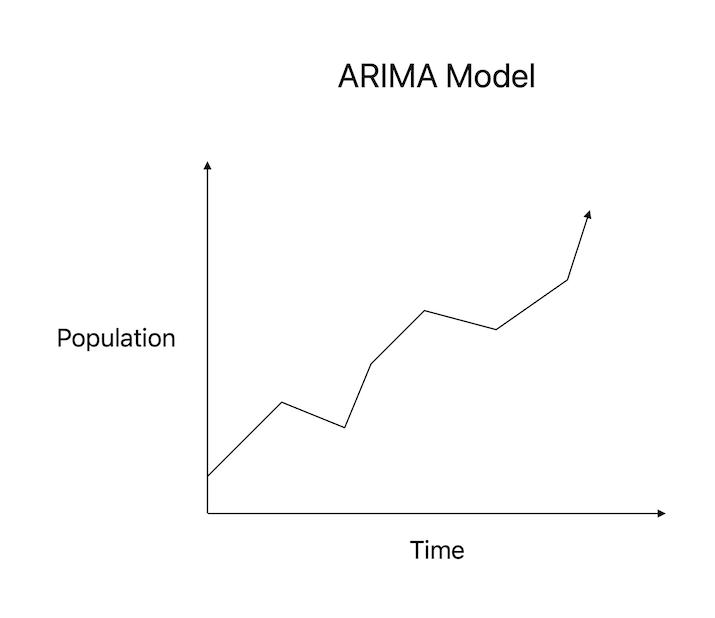# ARIMA model coefficient condition explained

In order to predict future values using the data at hand, time series analysis frequently employs Autoregressive Integrated Moving Average (ARIMA) models. These models use the moving average and autoregressive coefficients to represent the link between past and future data. For the model to be trustworthy and accurate, it is crucial to comprehend the criteria for these coefficients. This blog article will look at the requirement for the ARIMA model coefficients and their importance.

## What are ARIMA Models?

ARIMA models are statistical time series data analysis models. They have three components: autoregressive (AR), integrated (I), and moving average (MA). The AR word represents the relationship between past and present values, whereas the MA term denotes the impact of random shocks or noise. The I term is used to represent the trend in the data by differencing the time series.

ARIMA models predict future values by predicting the coefficients of these variables using historical data. The accuracy of these coefficients is crucial to the model's validity and reliability. Further down, the condition for these coefficients is provided.

Here is a basic example of the ARIMA model.## Condition for ARIMA Model Coefficients

The ARIMA model coefficients must meet the requirement of stationary behavior. The term "stationarity" describes how consistently statistical quantities like mean and variance appear throughout time. A time series' statistical characteristics alters over time if it is non-stationary, making it challenging to describe how one variable affects another.

The time series must be differentiated until it becomes stationary if the ARIMA model coefficients are to be guaranteed to be stationary. To eliminate any trend or seasonality in the data, differencing is a technique that includes subtraction of the current value from the prior value. The ARIMA model's coefficients can be precisely determined once the time series has reached a stationary state.

## Significance of the Condition for ARIMA Model Coefficients

To guarantee the model's accuracy and validity, the criterion for ARIMA model coefficients must be met. Inaccurate estimates from the model might result in poor decision-making if the coefficients are non-stationary. Spurious regression, which happens when a link between two variables is found when there is actually none, can also be caused by non-stationary coefficients.

Moreover, the need for the ARIMA model coefficients guarantees that the model is resistant to any changes in the data. For the model to provide reliable projections, the data must be flexible enough to accommodate changes over time. The model is stable and can make accurate predictions even when the data changes because of stationary coefficients.

## How to Check the Stationarity of ARIMA Model Coefficients?

The Augmented Dickey-Fuller (ADF) test can be applied to check the stationary properties of the coefficients in the ARIMA model. The ADF test, a statistical procedure, is used to detect whether or not a time series is stationary. The null hypothesis is examined by looking for a time series unit root, which would suggest that the series is non-stationary. The null hypothesis is rejected and the time series is determined to be stationary if the ADF test's p-value is less than the significance threshold, which is typically 0.05.

Another way to check if the ARIMA model coefficients are stationary is to plot the autocorrelation function (ACF) and partial autocorrelation function (PACF) of the differenced time series. The ACF measures the correlation between the current observation and its lagged values, whereas the PACF evaluates the correlation between the present observation and its lagged values after accounting for intervening data. Plots of the ACF and PACF that show a sharp decrease to zero suggest that the time series is likely stationary.

## Conclusion

In conclusion, to guarantee the model's correctness and validity, the criterion for ARIMA model coefficients is crucial. As long as the model's coefficients are stationary, accurate projections can be made even when the data is dynamic. ADF testing and visualizing the ACF and PACF of the differenced time series are two methods for determining if the ARIMA model coefficients are stationary. It is necessary to differentiate the time series until it becomes stationary if the coefficients are discovered to be non-stationary before estimating the ARIMA model coefficients.

Think about a farmer who is attempting to predict the price of corn for the upcoming year. You may use an ARIMA model to assess previous maize price data and take into account elements like weather, demand, and supply to forecast the price of maize in the future. By selecting the most advantageous period for planting, harvesting, and selling your products, you can boost your profits.

Another possibility is that you're a stock trader attempting to predict the price of a certain stock. You can use an ARIMA model to analyze historical stock prices and economic variables like interest rates and inflation to forecast what the price would be in the future. With this information, you can make better-informed investment decisions and even achieve substantial gains.

Updated on: 25-Apr-2023

508 Views